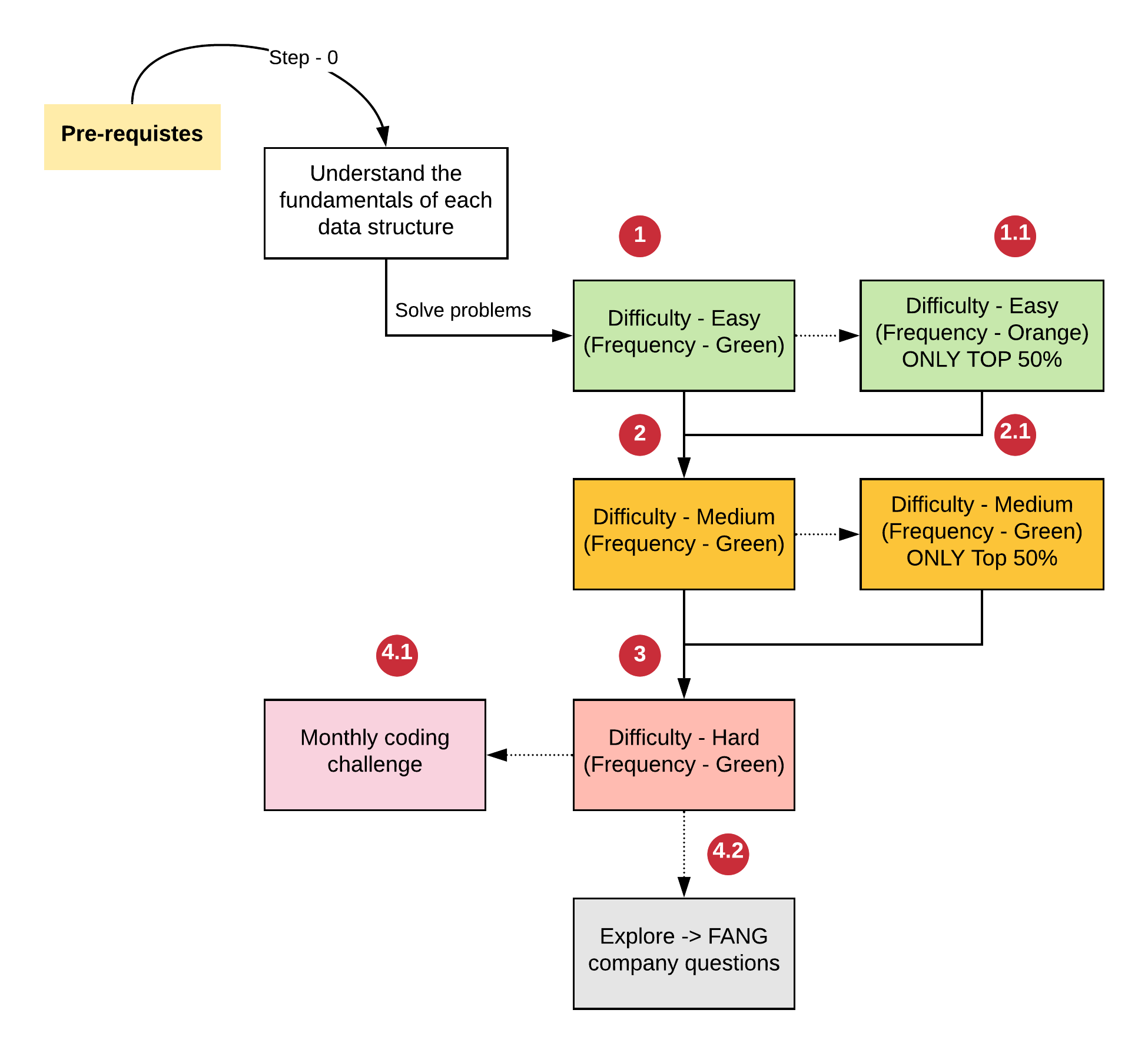1596429120

# What is a Palindrome?

A palindrome is a word that reads the same forwards and backwards. In my universe, there are two kinds of palindrome: “Odd Pal” and “Even Pal”.

## Even Pal

Even Pal is a palindrome which has an even length, for example: “ZYXXYZ”. In the visualization below you can see how we can split it into 2 parts that have size 3: “ZYX” and “XYZ” . This palindrome will always have two centres called Left Center” at index2 and “Right Center” at index3.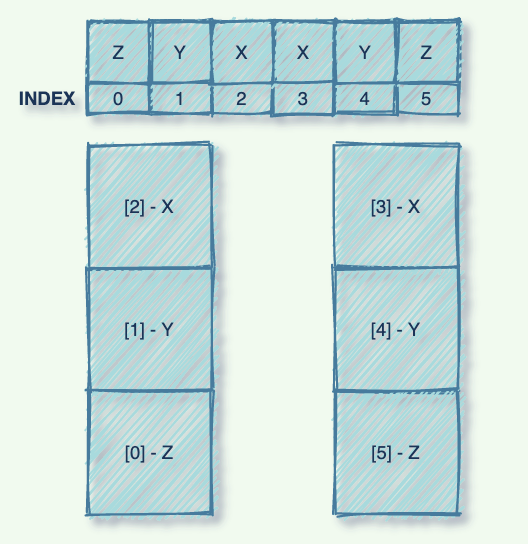“Even Pal”

Notice that:

• character at index2 = character at index3 (mirror 1)
• character at index1 = character at index4 (mirror 2)
• character at index0 = character at index5 (mirror 3)

It is because of the above equality of characters at their respective indexes that the string is a palindrome and the two parts look like a sequence of 3 mirrors.

## Odd Pal

Odd Pal is a palindrome which has an odd length, example: “ZYXWXYZ”. In the visualization below you can see how we can split the string into 2 parts that have size 3: “ZYX” and “XYZ”, while “W” at index3 becomes the center.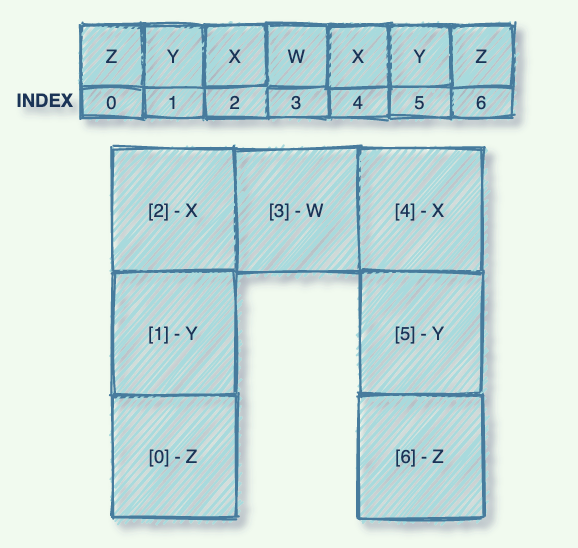“Odd Pal”

Notice that:

• character at index2 = character at index4 (mirror 1)
• character at index1 = character at index5 (mirror 2)
• character at index0 = character at index6 (mirror 3)

Notice how again the two parts look like a sequence of 3 mirrors.

# Problem

The problem states that we have to find the longest substring that is a palindrome in the string. A substring is a section of a string that consists of contiguous elements. Some examples are:

Input1: “BABAD” | Output1: “BAB” or “ABA”

Input2: “CBBD” | Output2: “BB”

Input3: “A” | Output3: “A”

# Solutions

## O(n²) Complexity

The simplest way to solve for this is to take every index as 1) a center 2) a Left Center and expand outwards to make character comparisons and find mirrors. The sequence with the maximum number of mirrors would be returned. Seems very simple and intuitive but the complexity is O(n²) because expanding a palindrome around a center could take O(n) time and we will explore every index in the string to be a center and left center, so O(n²) would be the time complexity.

## O(n) Complexity

We can improve this runtime if we think of a way where we can leverage the previous palindromes found (as we navigate through the string) and reduce the “expanding outwards” part. Luckily, this is possible with “Manachar’s algorithm”.

# Manachar’s algorithm

Before diving into the algorithm itself, we need to learn a few things first. For simplicity we will be focusing on Odd Pals for the most part. So let’s learn a few things in order:

#palindrome #leetcode #algorithms #substring #coding #algorithms

## Buddha Community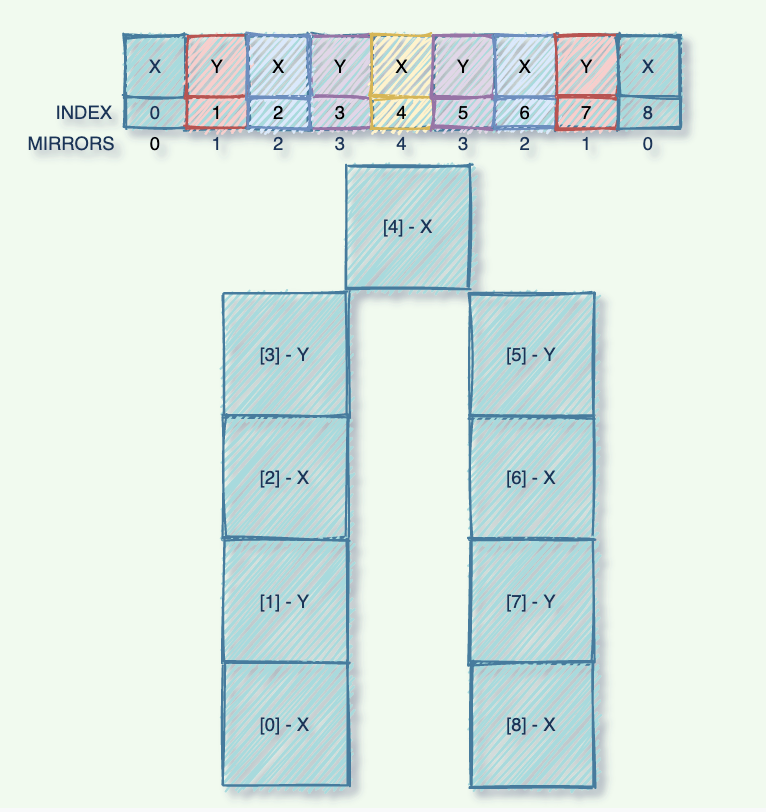1596429120

# What is a Palindrome?

A palindrome is a word that reads the same forwards and backwards. In my universe, there are two kinds of palindrome: “Odd Pal” and “Even Pal”.

## Even Pal

Even Pal is a palindrome which has an even length, for example: “ZYXXYZ”. In the visualization below you can see how we can split it into 2 parts that have size 3: “ZYX” and “XYZ” . This palindrome will always have two centres called Left Center” at index2 and “Right Center” at index3.“Even Pal”

Notice that:

• character at index2 = character at index3 (mirror 1)
• character at index1 = character at index4 (mirror 2)
• character at index0 = character at index5 (mirror 3)

It is because of the above equality of characters at their respective indexes that the string is a palindrome and the two parts look like a sequence of 3 mirrors.

## Odd Pal

Odd Pal is a palindrome which has an odd length, example: “ZYXWXYZ”. In the visualization below you can see how we can split the string into 2 parts that have size 3: “ZYX” and “XYZ”, while “W” at index3 becomes the center.“Odd Pal”

Notice that:

• character at index2 = character at index4 (mirror 1)
• character at index1 = character at index5 (mirror 2)
• character at index0 = character at index6 (mirror 3)

Notice how again the two parts look like a sequence of 3 mirrors.

# Problem

The problem states that we have to find the longest substring that is a palindrome in the string. A substring is a section of a string that consists of contiguous elements. Some examples are:

Input1: “BABAD” | Output1: “BAB” or “ABA”

Input2: “CBBD” | Output2: “BB”

Input3: “A” | Output3: “A”

# Solutions

## O(n²) Complexity

The simplest way to solve for this is to take every index as 1) a center 2) a Left Center and expand outwards to make character comparisons and find mirrors. The sequence with the maximum number of mirrors would be returned. Seems very simple and intuitive but the complexity is O(n²) because expanding a palindrome around a center could take O(n) time and we will explore every index in the string to be a center and left center, so O(n²) would be the time complexity.

## O(n) Complexity

We can improve this runtime if we think of a way where we can leverage the previous palindromes found (as we navigate through the string) and reduce the “expanding outwards” part. Luckily, this is possible with “Manachar’s algorithm”.

# Manachar’s algorithm

Before diving into the algorithm itself, we need to learn a few things first. For simplicity we will be focusing on Odd Pals for the most part. So let’s learn a few things in order:

#palindrome #leetcode #algorithms #substring #coding #algorithms1624874940

## Leetcodes Algorithm Series: Contains Duplicate

Hello, hello! As I continue my job search, I am practicing my algorithm questions on Leetcode. So, I thought I would blog about some Leetcode problems as I solve them.

As a Bootcamp graduate, I did not get much practice in algorithms, so it has been a very fun and sometimes frustrating trial and error in recognizing patterns, optimizing my code to be faster (looking at you Big-O), learning how to break down a problem, and sometimes implementing neat math tricks to solve these algorithm problems. I have been practicing them using JavaScript.

As I’m relatively new to algorithm problems, I’ve started out with their Easy Collection of Top Interview Questions. So in Contains Duplicate:

Given an integer array `nums`, return `true` if any value appears at least twice in the array, and return `false` if every element is distinct.

Examples:

``````Input: nums = [1,2,3,1]
Output: true

Input: nums = [1,2,3,4]
Output: false
Input: nums = [1,1,1,3,3,4,3,2,4,2]
Output: true
``````

Simply, if any numbers in the array appear more than once in the array, I need to return `true` . Now, I will definitely need to figure out a way to compare a number to the other numbers. Additionally, I will most likely need to loop through the array so I have access to each number to then compare it to others.

First, I thought that I could iterate through the array, and at each instance, I iterate through another loop of the remaining integers and compare them to see if any are equal (===). However, per my beginner understanding of Big-O, this would yield me an unfavorable time complexity of O(n²).

Then, I thought that I could create an object and count how many times a number in the array appears. By doing this, I could then check to see if any of the numbers had a count greater than 1. If so, then I could return `true` else I would return `false` .

#computer-science #leetcode #javascript #programming #algorithms #leetcodes algorithm1597507280

## Longest substring whose characters can be rearranged to form a Palindrome

A Computer Science portal for geeks. It contains well written, well thought and well explained computer science and programming articles, quizzes and practice/competitive programming/company interview Questions.

#bit magic #mathematical #strings #palindrome #substring #algorithms1594945380

# Problem

Given a string, find the length of the longest sub-string without repeating characters.

# Examples

## Example 1

``````Input: "abcabcbb"
Output: 3
Explanation: The answer is "abc", with the length of 3.
``````

## Example 2

``````Input: "bbbbb"
Output: 1
Explanation: The answer is "b", with the length of 1.
``````

## Example 3

``````Input: "pwwkew"
Output: 3
Explanation: The answer is "wke", with the length of 3\. Note that the answer must be a sub-string, "pwke" is a sub-sequence and not a sub-string.
``````

# A note on Strings in Rust

One thing to note is that Rust does not implement traditional string indexing due to issues that come up with utf-8 encodings. There are a few approaches to get characters out of a string, but I’ll just convert the input string to a vector of chars. It’s an interesting topic, but out of scope for this article.

# Approach 1: Brute Force

A naive solution to this problem is to iterate through every possible sub-string and track the length of the longest sub-string seen so far.

# Algorithm

This approach can be separated into two function. One function should handle iterating over the sub-strings and tracking the length of the longest sub-string. The other function should handle check for uniqueness.

## Sub-string Iteration Algorithm

To iterate over the sub-strings, this approach uses two loops. The outer loop iterates from `i` to `n` where `n` is the length of the input string. The inner loop iterates from `i` + 1 to `n` inclusively.

The algorithm looks like this:

``````initialize n to length of string
initialize length to 0
for i = 0 to n
for j = i + 1 to n + 1
if sub-string of characters between i and j are unique
update length if j - i is greater than current length
return length
``````

## All Unique Algorithm

To check if a sub-strings characters are all unique, this function will add each character to a set. If a duplicate character is found the function will return false, otherwise it will return true.

This algorithm looks like this:

``````initialize a HashSet of char
initialize a vector of chars
for i = start to end
if the char exists in the set
return false
else
return true
``````

# Implementation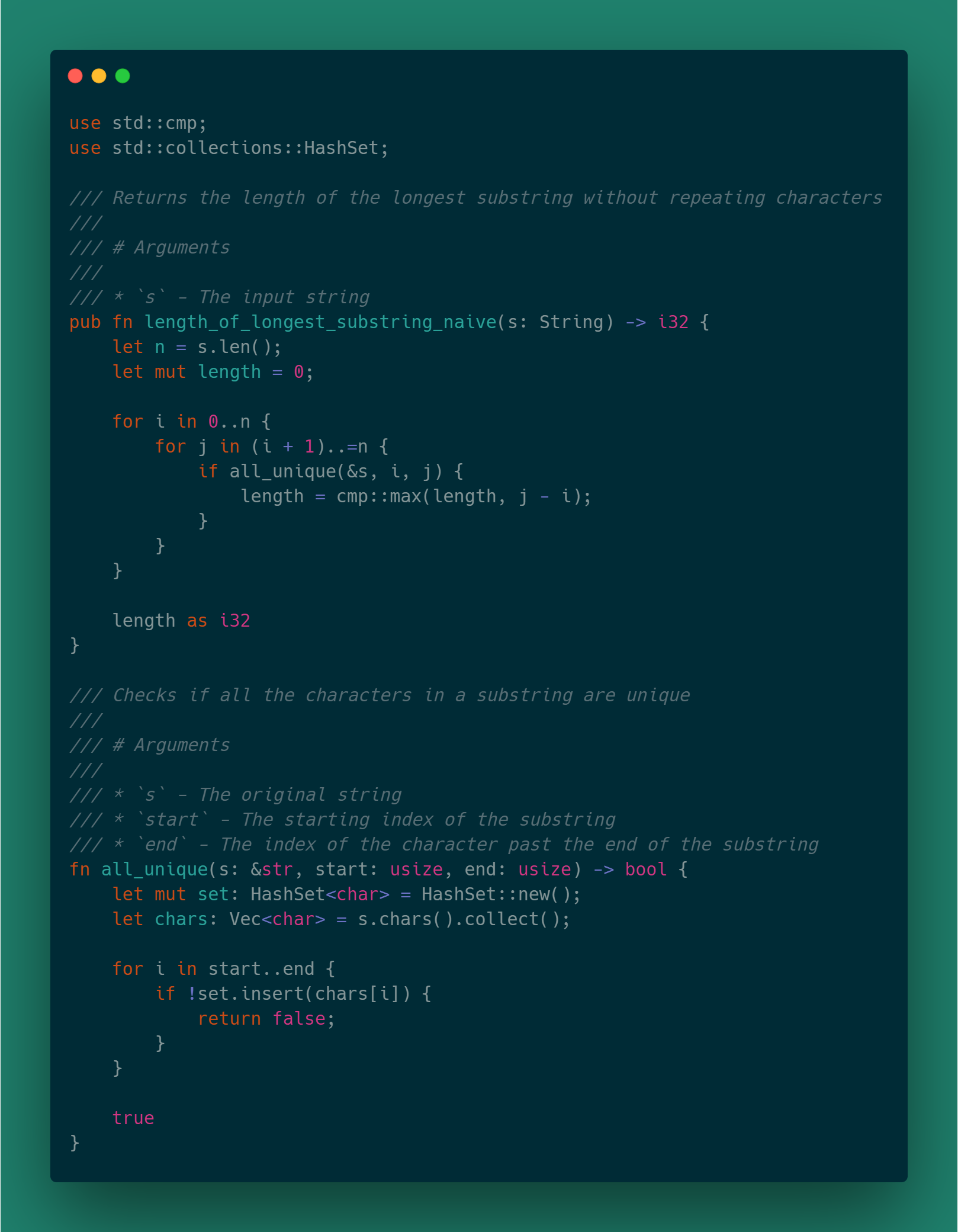#programming-problem #rust #algorithms #leetcode #algorithms1596487260

# What is LeetCode.com?

It’s a website where people–mostly software engineers–practice their coding skills. It’s pretty similar to sites like HackerRank & Topcoder which will rank your code written for a particular problem against the ones submitted by other users

# People who use them

Leetcode gets primarily used for two use cases:

# Purpose of this post

I never had a clear understanding when it comes to data structures and algorithms, so being a software engineer for the last 12 years, I thought it’s not a bad idea to at least start now. So as of Dec 2019, I became a premium member in Leetcode and committed myself to get as much value out of 150\$ that I spent on the membership fees. Leetcode is like an ocean; it has closer to 2000+ questions, which keeps growing day by day.

In fact, this was my exact reaction when I open the website for the first time after looking at the number of questions and categories it hadIn this post, let me explain some of the methods I developed over some time, which helped me to understand and learn about these data structures & algorithms.

# Get the basics right — Don’t run too fastMost people new to Leetcode directly jump into solving the problems without getting a proper understanding of the underlying data structures like a linked liststack, queue, tree, etc. Trust me this is a bad idea

I would recommend spending some time understanding these data structures, which are the building blocks for solving any problems.

Even if you are not able to go through all the data structures, at least get a strong understanding of the linked list and _binary trees. _Fortunately, there are some excellent materials created by Standford, which can help you with this:

## Binary tree